Courses

# Unit & Dimensions Vectors(Part- 3) - Physics, Solution by DC Pandey NEET Notes | EduRev

## DC Pandey (Questions & Solutions) of Physics: NEET

Created by: Ciel Knowledge

## NEET : Unit & Dimensions Vectors(Part- 3) - Physics, Solution by DC Pandey NEET Notes | EduRev

The document Unit & Dimensions Vectors(Part- 3) - Physics, Solution by DC Pandey NEET Notes | EduRev is a part of the NEET Course DC Pandey (Questions & Solutions) of Physics: NEET.
All you need of NEET at this link: NEET

Section-II
Subjective Questions

Ques 1: Young’s modulus of steel is 2.0 x 1011 N / m2. Express it in dyne/cm2.
Ans: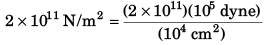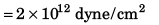Ques 2: Surface tension of water in the CGS system is 72 dynes/cm . What is its value in SI units?
Ans: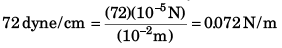Ques 3: In the expression y = a sin (ωt + θ), y is the displacement and t is the time. Write the dim ensions of a, ω and θ.
Ans: [a] = [y] = [L]
Sol: [wt] = [M0L0 T0] ∴ [ω] = [T-1]

[θ] = [M0L0 T0]

Ques 4: The relation between the energy E and the frequency v of a photon is expressed by the equation E = hv, where h is Planck’s constant. Write down the SI units of h and its dimensions.
Ans: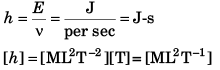Ques 5: Write the dimensions of a and b in the relation.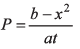where P is power, x is distance and t is time.
Ans: [b] = [x2] = [L2]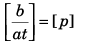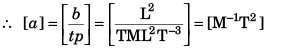Ques 6: Check the correctness of the relation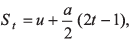where u is initial velocity, a is acceleration and St is the displacement of by the body in tth second.
Ans: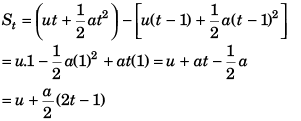Here t in second. Hence the given equation seems to be dimensionally incorrect. But it is correct because 1 is hidden.

Ques 7: Let x and a stand for distance.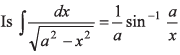dim ensionally correct?
Ans: LHS is dimensionless. While RHS has the dimensions [L-1].

Ques 8: In the equation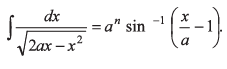Find the value of n.
Ans: LHS is dimensionless. Hence n = 0.

Ques 9: Show dimensionally that the expression,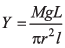is  dimensionally correct, where  Y is Young’s modulus of the material of wire, L is length of wire, Mg is the weight applied on the wire and l is the increase in the length of the wire.
Ans: Just write the dimension of different physical quantities.

Ques 10: The energy E of an oscillating body in simple harmonic motion depends on its mass m, frequency n and amplitude a. Using the method of dimensional analysis find the relation between E, m, n and a.
Ans: E = kmxnyaz.

Here k = a dimensionless constant
∴ [E] = [m]x [n]y [a]z
∴ [ML2 T–2] = [M]x[T–1]y[L]z
∴ x = 1, y = 2 and z = 2

Ques 11: The centripetal force F acting on a particle moving uniformly in a circle may depend upon mass (m), velocity (v) and radius r of the circle. Derive the formula for F using the method of dimensions.

Ans: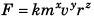(k = a dimensionless constant)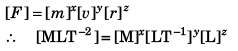Solving we get,
x = 1, y = 2 and z = - 1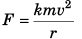Ques 12: Taking force F, length L and time T to be the fundamental quantities, find the dimensions of (a) density, (b) pressure, (c) momentum and (d) energy.
Ans: [d] = [F]x [L]y [T]z
∴ [ML–3] = [MLT–2]x[L]y[T]z
Equating the powers we get,
x = 1, y = - 4, z = 2
∴ [ d] = [FL–4 T2]
Similarly other parts can be solved.

Vectors

Ques 13: Find the cosine of the angle between the vectors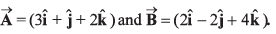Ans: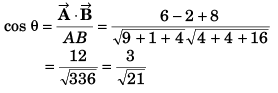Ques 14: Obtain the angle between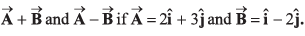Ans: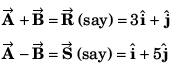Angle between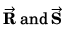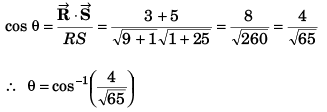Ques 15: Under what conditions will the vectors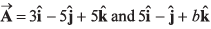be perpendicular to each other ?
Ans: Their dot product should be zero.

Ques 16: Deduce the condition for the vectors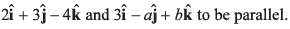Ans: Ratio of coefficients of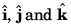should be same.

Ques 17: Three vectors which are coplanar with respect to a certain rectangular co-ordinate system are given by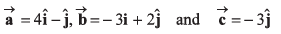Find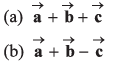(c) Find the angle between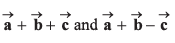Ans: No solution is required.

Ques 18: Find the components of a vector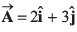along the directions of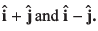Ans: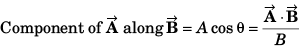Ques 19: If vectors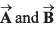be respectively equal to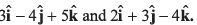Find the unit vector parallel to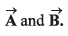Ans: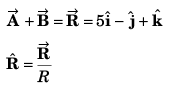Ques 20: If two vectors are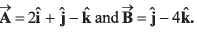By calculation, prove that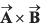is perpendicular to both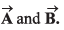Ans: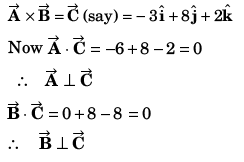Ques 21: Find the area of the parallelogram whose sides are represented by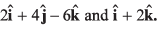Ans: Area of parallelogram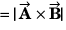Ques 22: The resultant of two vectors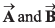is at right angles to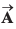and its magnitude is half of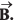Find the angle between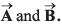Ans: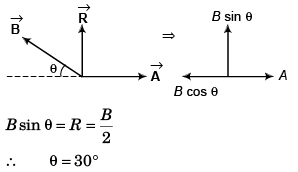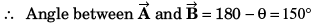Ques 23: The x and y-components of vectorare 4 m and 6 m respectively. The x and y-components of vector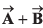are 10 m and 9 m respectively. Calculate for the vector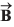the following
(a) its x andy-components
(b) its length
(c) the angle it makes with x-axis
Ans: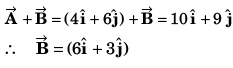Ques 24: Prove by the method of vectors that in a triangle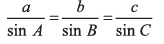Ans: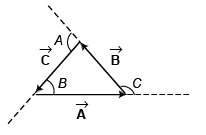Applying sine law, we have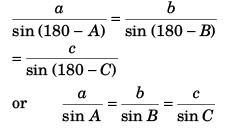Ques 25: Four forces of magnitude P, 2P, 3P and AP act along the four sides of a square ABCDm cyclic order. Use the vector method to find the resultant force.
Ans: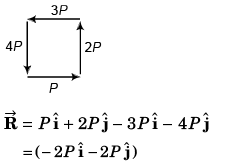Ques 26: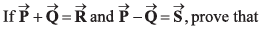R2 + S2 = 2(P2 + Q2)
Ans: R2 = P2 + Q2 + 2PQ cos θ
S2 = P2 + Q2 - 2PQ cos θ
∴ R2 + S2 = 2 (P2 + Q2)

Offer running on EduRev: Apply code STAYHOME200 to get INR 200 off on our premium plan EduRev Infinity!

210 docs

,

,

,

,

,

,

,

,

,

,

,

,

,

,

,

,

,

,

,

,

,

,

,

,

;